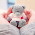Dear students,Quantitative Aptitude for SBI Clerk Prelims 2018

Today is the Day 54 of the SBI Clerk 60 Days Study PlanNumerical Ability Section has given heebie-jeebies to the aspirants when they appear for a banking examination. As the level of every other section is only getting complex and convoluted, there is no doubt that this section, too, makes your blood run cold. The questions asked in this section are calculative and very time-consuming. But once dealt with proper strategy, speed, and accuracy, this section can get you the maximum marks in the examination. Following is the Numerical Ability quiz to help you practice with the best of latest pattern questions.

Q1. CI on a certain sum for 2 years is Rs. 40.80 and SI is Rs. 40 for same years. What will be the rate of interest?
(a)2%
(b)5%
(c)4%
(d)8%
(e)12%

Q2. A tree’s height increases annually by 1/8th of its height. By how much will its height increase after 2.5 years, if it stands today at 10 m height?
(a)3 m
(b)3.44 m
(c)3.6 m
(d)3.88 m
(e)4 m

Q3. Siddhartha has deposited certain amount in the bank to earn compound interest at    10% per annum. The difference between the interest of third year and second year on the amount is Rs. 110. What amount has Siddhartha deposited?
(a)Rs.8500
(b)Rs.10,000
(c)Rs.9500
(d)Rs.11,000
(e) Rs.12000

Q4. Simple interest on a certain sum at the rate of 12% per annum for 3 years is Rs. 3024. What is the compound interest at the same sum at the rate of 20% for two years?
(a) Rs. 3850
(b) Rs 3696
(c) Rs 3966
(d) Rs 3669
(e) None of these

Q5. A bike dealer gives a discount of 20% on marked price of bikes. Ajit purchases a bike in Rs. 50,500. What is the marked price of bike?
(a) Rs. 60,500
(b) Rs. 64,225
(c) Rs 63,125
(d) Rs 65,500
(e) None of these

Solutions (1-5):

Q6. Pragya gives a certain sum to Anamika on simple interest in such a way that her money will be double in 8 years. But after 5 years Anamika returned the money and paid Rs. 2000 as interest. Find the principle amount.
(a) Rs. 4000
(b) Rs. 3200
(c) Rs. 3600
(d) Rs. 6000
(e) Rs. 5600

Q7. Arun took a loan of Rs. 16000 with simple interest for as many years as the rate of interest. If he paid Rs. 7840 as interest at the end of the loan period, what was the rate of interest.
(a) 8% p.a.
(b) 12% p.a.
(c) 5% p.a.
(d) 7% p.a.
(e) 9% p.a.

Q8. A book was sold at Rs. 165 at a profit of 10%. If it were bought at Rs. 30 less and sold at Rs. 15 more, then find the new profit percentage.
(a) 50%
(b) 40%
(c) 60%
(d) 54%
(e) 46%

Q9. Madhu invested Rs. 15000 in two schemes A & B. Scheme A gives 8% SI P.A. and scheme B gives 13% SI. P.A. If Madhu received Rs. 3000 as total interest from both schemes after two years. Then find the amount invested in scheme B.
(a) Rs. 7000
(b) Rs. 9000
(c) Rs. 6000
(d) Rs. 5000
(e) Rs. 8000

Q10. The ratio of CI and SI for two years is 3 : 2 while  sum invested is Rs. 5000. What will be the difference between the CI and SI earned after three years?
(a)Rs. 15,000
(b) Rs. 20,000
(c)Rs. 10,000
(d)Rs. 25,000
(e)None of these

Solutions (6-10):

Directions (11-15): Find the value of (?) in the given number series.

Q11.  2, 4, 12, 48, 240, ?
(a) 960
(b) 1440
(c) 1080
(d) 1920
(e) 1640

Q12.  7, 26, 63, 124, 215, 342, ?
(a) 481
(b) 511
(c) 391
(d) 421
(e) 728

Q13.  8, 24, 12, 36, 18, 54, ?
(a) 27
(b) 108
(c) 68
(d) 72
(e) 162

Q14.  15, 31, 63, 127, 255, ?
(a) 513
(b) 511
(c) 517
(d) 523
(e) 515

Q15.  11, 13, 17, 19, 23, 29, 31, 37, 41, ?
(a) 42
(b) 47
(c) 53
(d) 51
(e) 43

Solutions (11-15):

S11. Ans.(b)
Sol. Go on multiplying the given numbers by 2, 3, 4, 5, 6. So, the correct next number is 1440.

S12. Ans.(b)
Sol. Number are (2³ – 1), (3³ – 1), (4³ – 1), (5³ – 1), (6³ – 1), (7³ – 1) etc.
So, the next number is (8³ – 1) = (512 – 1) = 511.

S13. Ans.(a)
Sol. Numbers are alternately multiplied by 3 and divided by 2.
So, the next number = 54 ÷ 2 = 27.

S14. Ans.(b)
Sol. Each number is double the preceding one plus.
So, the next number is (255 × 2) + 1 = 511.

S15. Ans.(e)

Sol. Numbers are all primes. The next prime is 43.#### 1 comment:

1.answers are not visible